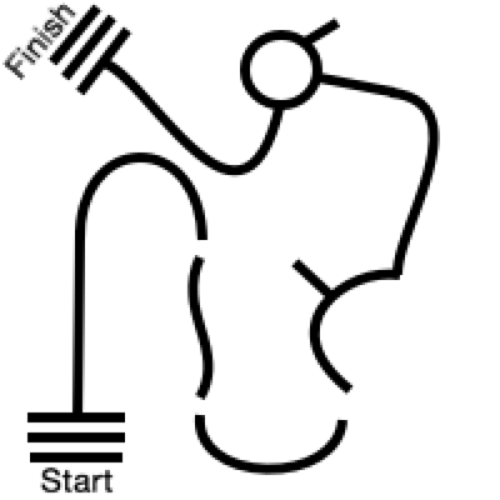#### Howdy, Stranger!

We are about to switch to a new forum software. Until then we have removed the registration on this forum.

# Translating/Rotating/Camera in P3D

edited March 2016

Good morning world,

I'm getting foiled by P3D and translating/rotating and how they work with camera(), but I'm learning a bit about how they work. So far I've learned that calling camera() with the specified values AFTER using translate/rotate earlier in the program causes undesirable results, I'm guessing because once compiled it is run first as actual translate/rotate. This can get messy when trying to take snapshots of a 3D scene. I've since started looking into beginCamera() and endCamera(), and boy are those interesting. One thing that I've learned about the camera using beginCamera() and endCamera() is that changes to the camera (matrix?) are ADDED instead of set. This is fine, but I'm still finding that the changes to the camera aren't happening when the changes are called. I've created this little sketch to try and showcase my headache, comment in and out each box(150) to see different results:

``````void setup(){
size(500,500,P3D);
}
void draw(){
background(0);
stroke(150);
noFill();

sphere(60);
line(0,0,0,0,-100,0);

//box(150);          //Enable/disable these one by one

beginCamera();
camera();
translate(width/2,height/2);
endCamera();

box(150);          //Enable/disable these one by one

beginCamera();
endCamera();

//box(150);          //Enable/disable these one by one
box(20);
line(0,0,0,0,-100,0);
}
``````

I'm wondering if anyone has a better explanation (through code examples preferably) regarding rotating and translating within a 3D space, and how the camera function alters the prior translates and rotates. I have some experience with using push/popMatrix() and translate/rotate in 2D land, but I'm not sure how push/popMatrix() affect the camera matrix, and finally how this all plays with the main window pixels.

Finally, I will talk briefly about what I'm actually trying to do. I'm using processing to create a 3D robot simulation environment (for line following algorithm testing) and I would like this sketch to output a 2D image of the robot's perspective. The problem I am running into is when I call translate/rotate to move about the scene and place the robot/floor etc, I still have to call camera() with the appropriate values given to me by the position of the robot to look at the world from the perspective of the robot. I was having lots of trouble doing it this way when I realized that camera() stuff must be done first before moving about and drawing. The way that I was trying to grab the 2D image was by reorienting the camera to the desired position, grabbing the window pixels and giving them to a PImage, then reorienting the camera to a top-down perspective. The problem with this was the the Image only showed the final position of the camera, not any of the intermediary steps. Here's the actual sketch that I'm working on, comment in/out the image at the end of main to see what the current image output would be (use arrow keys to drive around)(I attached TestCourseLine.png I think):

``````//  Simulation environment for testing line following

int windowWidth = 1000;
int windowHeight = 700;

PImage course;
PImage robotCam;
PImage roomCam;
int robotCamWidth = 600;
int robotCamHeight = 400;
int window[][];
int robotCamArray[][];

//Camera (independent of translations)
float cameraX = 500;
float cameraY = 350;
float cameraZ = 606;
float cameraCenterX = 500;
float cameraCenterY = 350;
float cameraCenterZ = 0;
float targetDistance = 0;

int robotStep = 5;
float robotTurn = .1;

//Robot Position/orientation relative to center origin
float robotX = -170;
float robotY = 290;
float robotZ = 25;
float robotRotZ = 4.7;

//RobotCam position/orientation relative to robot
int robotCamX = 20;
int robotCamY = 0;
int robotCamZ = 30;
float robotCamRotX = 0;
float robotCamRotZ = 0;
float robotCamRotY = 0.4;

boolean moveRobot = true;

boolean chaseCam = false;

void setup(){

size(windowWidth,windowHeight,P3D);

frameRate(10);

window = new int[windowWidth][windowHeight];
robotCamArray = new int[robotCamWidth][robotCamHeight];

robotCam = createImage(robotCamWidth,robotCamHeight, RGB);

}
void draw(){

pushMatrix();
//Move the window camera to the robot's camera (Must be done before other translations/rotates)
cameraX = cos(robotCamRotZ+robotRotZ)*0+(robotX+width/2);
cameraY = sin(robotCamRotZ+robotRotZ)*0+(robotY+height/2);
cameraZ = robotCamZ+robotZ;
targetDistance = (robotZ+robotCamZ)/sin(robotCamRotY);
cameraCenterX = cos(robotCamRotZ+robotRotZ)*targetDistance+(robotX+width/2);
cameraCenterY = sin(robotCamRotZ+robotRotZ)*targetDistance+(robotY+height/2);
camera(cameraX, cameraY, cameraZ, cameraCenterX, cameraCenterY, cameraCenterZ, 0,0,-1);

background(0);

fill(100);
stroke(200);
noFill();

pushMatrix();
//Center origin to scene
translate(width/2,height/2);

box(500);

//Create Floor
beginShape();
texture(course);
vertex(-250,-250,0,0,0);
vertex(250,-250,0,500,0);
vertex(250,250,0,500,500);
vertex(-250,250,0,0,500);
endShape(CLOSE);

//Draw Robot and camera
pushMatrix();
translate(robotX, robotY, robotZ);
rotateZ(robotRotZ);
box(50);
//line(0,0,0,100,0,0);
translate(robotCamX,robotCamY,robotCamZ);
rotateX(robotCamRotX);
rotateZ(robotCamRotZ);
rotateY(robotCamRotY);
fill(100);
box(20);
line(0,0,0,30,0,0);
popMatrix();

//Load the pixels of the last camera perspective and the new image

//Load the window pixels into an array for easy access
for (int i = 0; i < pixels.length; i++){
window[i-((i/windowWidth)*windowWidth)][i/windowWidth] = int(brightness(pixels[i]));
}

//Give the new image the pixel data from the last camera perspective
for (int y = 0; y < robotCamHeight; y++){
for (int x = 0; x < robotCamWidth; x++){
robotCamArray[x][y] = window[x+(windowWidth-robotCamWidth)/2][y+(windowHeight-robotCamHeight)];
}
}

//Load the robotCamArray data into robotCam pixels
for (int i = 0; i < robotCam.pixels.length; i++){
robotCam.pixels[i] = color(robotCamArray[i-((i/robotCamWidth)*robotCamWidth)][i/robotCamWidth]);
}
updatePixels();
robotCam.updatePixels();
popMatrix();
//popMatrix();

popMatrix();

//background(0);

translate(width/2,height/2,100);

//image(robotCam,-robotCamWidth/2,-robotCamHeight/2,robotCamWidth,robotCamHeight);

}

void keyPressed(){

if (key == 'm'){
moveRobot = true;
} else if (key == 'c'){
moveRobot = false;
} else if (key == 'w'){
moveRobot = false;
}

if (moveRobot == true){
if (key == CODED){
switch(keyCode){
case UP:
robotX += cos(robotRotZ)*robotStep;
robotY += sin(robotRotZ)*robotStep;
break;
case DOWN:
robotX += cos(robotRotZ)*-robotStep;
robotY += sin(robotRotZ)*-robotStep;
break;
case LEFT:
robotRotZ -= robotTurn;
break;
case RIGHT:
robotRotZ += robotTurn;
break;
}
}
} else if (adjustRobotCam == true){
if (key == CODED){
switch(keyCode){
case UP:
robotCamRotY -= robotTurn;
break;
case DOWN:
robotCamRotY += robotTurn;
break;
case LEFT:
robotCamRotZ -= robotTurn;
break;
case RIGHT:
robotCamRotZ += robotTurn;
break;
}
}
} else if (adjustWindowCam == true){
if (key == CODED){
switch(keyCode){
case UP:
//camera -= robotTurn;
break;
case DOWN:
robotCamRotY += robotTurn;
break;
case LEFT:
robotCamRotZ -= robotTurn;
break;
case RIGHT:
robotCamRotZ += robotTurn;
break;
}
}
}
}
``````Tagged: